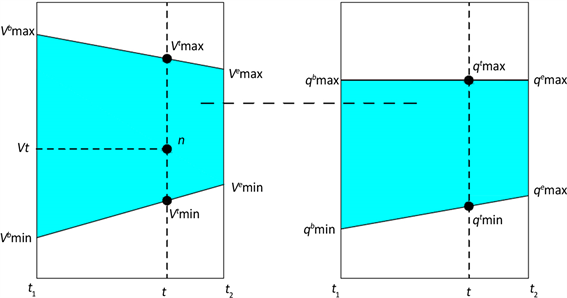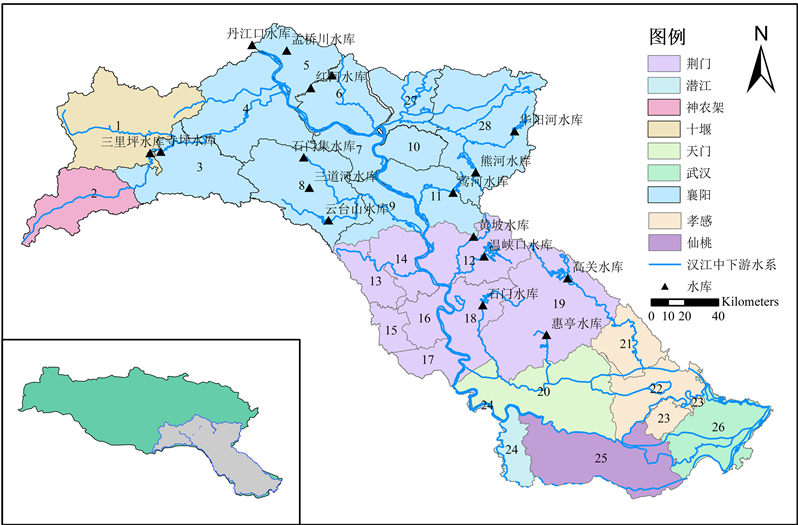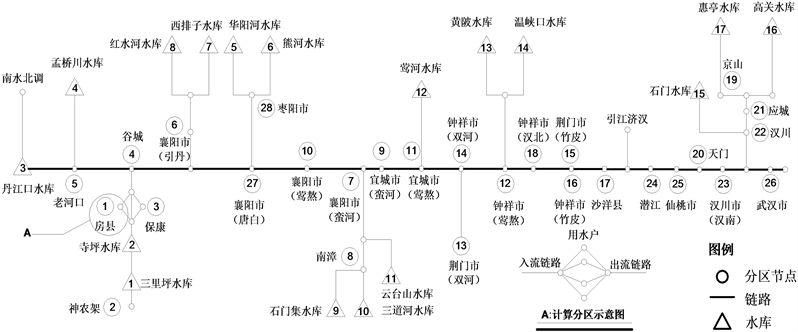﻿ 不同时间尺度下水资源配置效应

# 不同时间尺度下水资源配置效应Effects of Time Scales on Water Resources Allocation

Abstract: The water resources allocation is an important means to solve the problem of uneven distribution of water resources in time and space. However, there are few studies on the effects of time scales on water resources allocation. The effects caused by the variation of time scale are often ignored and lead to underestimation of water shortage risks in water resources management. IRAS water resources allocation model which can adjust the time step flexibly to adapt to different time scales, is employed to the middle and lower reaches of the Hanjiang River basin as a case study. There are three types of time scales (year, season and month) to be chosen for discussing the effects of time scales on water resources allocation. The results show that the effects of time scales on water resources allocation mainly depend on water resources conditions and the regulating capacity of the water system. Water shortage is few to find in all three-time scales if the water resources are enough to satisfy the water demand. However, the effects of time scales on water resources allocation are more sensitive in areas with water shortage and weak regulating capacity of the water system. As the variability of water inflow and demand water can be smoothed at long-term scale, the water shortage ratio at long-term scale is lower than that at a short-term scale. The results of the study have not only analyzed the effects of time scales on water resources allocation, but also warned the risks of water resources management at different time scales.

1. 引言

2. IRAS模型结构与原理

IRAS是一款能够灵活调整计算时间步长，适用于多种时间尺度条件的水资源配置模拟模型，目前该模型已经在英国泰晤士河流域、墨西哥瓜纳华托河流域与葡萄牙瓜迪亚纳河流域等地区得到了成功应用。模型以物理水网为基础，通过分析水资源系统中供、用、耗、排等主要环节中所涉及的要素和相互连接关系，结合水资源系统的来水序列、需水序列、各类参数以及约束条件，在不同的调度运行规则下，模拟系统内水资源的配置过程。其原理如图1所示：Figure 1. Framework of the IRAS model

2.1. 系统概化

IRAS模型水资源系统中所涉及的实体元素可以分为节点与有向线段两种类型。节点用于描述研究区域中的工程设施、控制结构与用水单元，根据实体元素的属性可将其分为水源节点、来水节点、用水节点、分流节点、汇流节点。有向线段用于描述节点之间存在的水量传输与影响关系，根据水流是否能够双向流动可将其分为双向线段与单向线段。其中单向线段又可进一步分为需水线段、分流线段、天然线段，参与到地表水的输送与分配过程之中；双向线段通常结合达西定律用于地下水的模拟。

2.2. 参数设置

2.3. 运行规则

1) 时间步长划分

IRAS模型以年为周期进行模拟计算，其时间步长可灵活调整，以适应不同时间尺度数据的输入，为在日、旬、月、季、年等时间尺度条件下水资源配置模拟计算提供了支持。每个时间步长可进一步划分为不同子时间步长(最小为1天)，子时间步长的数目越多，模拟过程越精细，配置结果精度越高，所需计算时间越长。

2) 缺水计算

${W}_{st}^{e}=\frac{\underset{1}{\overset{st}{\sum }}{W}_{st}^{in}-\underset{1}{\overset{st}{\sum }}{W}_{st}^{r,in}}{st-1}\ast \left(tst-st+1\right)$ (1)

${W}_{st}^{d}=\frac{{W}_{t}^{dem}-{W}_{t}^{dem}\ast f-\underset{1}{\overset{st-1}{\sum }}{W}_{st}^{in}-{W}_{st}^{e}}{tst-st+1}$ (2)

$E{W}_{st}^{k,out}=\underset{i=1}{\overset{N}{\sum }}{W}_{st}^{i,d}\ast {\alpha }_{st}^{i,k}$ (3)

3) 供水计算Figure 2. Calculation diagram of water supply discharge of the main reservoir

${P}_{t}=t/T$ (4)

${V}_{\mathrm{max}}^{t}={V}_{\mathrm{max}}^{b}\ast \left(1-{P}_{t}\right)+{V}_{\mathrm{max}}^{e}\ast {P}_{t}$ (5)

${V}_{\mathrm{min}}^{t}={V}_{\mathrm{min}}^{b}\ast \left(1-{P}_{t}\right)+{V}_{\mathrm{min}}^{e}\ast {P}_{t}$ (6)

${q}_{\mathrm{max}}^{t}={q}_{\mathrm{max}}^{b}\ast \left(1-{P}_{t}\right)+{q}_{\mathrm{max}}^{e}\ast {P}_{t}$ (7)

${q}_{\mathrm{min}}^{t}={q}_{\mathrm{min}}^{b}\ast \left(1-{P}_{t}\right)+{q}_{\mathrm{min}}^{e}\ast {P}_{t}$ (8)

${P}_{v}=\left({V}^{t}-{V}_{\mathrm{min}}^{t}\right)/\left({V}_{\mathrm{max}}^{t}-{V}_{\mathrm{min}}^{t}\right)$ (9)

${q}^{t}={q}_{\mathrm{min}}\ast \left(1-{P}_{v}\right)+{q}_{\mathrm{max}}\ast {P}_{v}$ (10)

4) 配水与耗水计算

2.4. 约束条件

1) 计算分区水量平衡约束

${W}_{st}^{i}=\underset{m=1}{\overset{M}{\sum }}{W}_{st}^{m}\cdot {\beta }_{st}^{i,m}+\underset{k=1}{\overset{K}{\sum }}{W}_{st}^{k,out}\cdot {\alpha }_{st}^{i,k}+{W}_{st}^{i,in}-\underset{j=1}{\overset{J}{\sum }}{W}_{st}^{i,j}\cdot {c}_{st}^{i,j}-{W}_{st}^{i,l}-{W}_{st}^{i,t}$ (11)

2) 水库约束

① 水库水量平衡约束

${V}_{st+1}^{k}={V}_{st}^{k}+{W}_{st}^{k,in}-{W}_{st}^{k,out}-{W}_{st}^{k,l}$ (12)

② 水库库容约束

${V}_{\mathrm{min}\text{}st}^{n}\le {V}_{st}^{n}\le {V}_{\mathrm{max}\text{}st}^{n}$ (13)

3) 非负约束

${W}_{st}^{i,j}\ge 0$ (14)

4) 需水约束

${W}_{st}^{i,j}\le D{W}_{st}^{i,j}$ (15)

5) 供水能力约束

$\underset{j=1}{\overset{J}{\sum }}{W}_{st}^{i,j}\le A{W}_{st}^{i}$ (16)

3. 汉江流域中下游地区水资源配置模型构建

3.1. 研究区——汉江流域中下游地区概况

3.2. 计算单元划分

3.3. 模型输入

1) 水平年及计算步长Figure 3. Spatial distribution of computing units and reservoirs in the middle and lower reaches of Hanjiang River basinFigure 4. Schematic diagram of the middle and lower reaches of Hanjiang River basin

2) 来水条件与需水条件Table 1. Annual off-stream water demand in the present and planning years (100 million m3)

3) 参数设置Table 2. Regression coefficient of every type of water use in the Hanjiang River basin

4. 结果分析

Table 3. Total water scarcity in the middle and lower reaches of Hanjiang River basin (10,000 m3)Table 4. Water scarcity in every city in the middle and lower reaches of Hanjiang River basin (10,000 m3)

5. 结论Figure 5. The process of water inflow and water demand in Jingmen city under seasonal and monthly scales

 王浩. 我国水资源合理配置的现状和未来[J]. 水利水电技术, 2006, 37(2): 7-14. WANG Hao. Current status and future trend of rational allocation of water resources in china. Water Resources and Hydropower Engineering, 2006, 37(2): 7-14. (in Chinese)

 尹家波, 郭生练, 吴旭树, 等. 两变量设计洪水估计的不确定性及其对水库防洪安全的影响[J]. 水利学报, 2018, 49(6): 715-724. YIN Jiabo, GUO Shenglian, WU Xushu, et al. Uncertainty of bivariate design flood estimation and its impact on reservoir flood prevention. Journal of Hydraulic Engineering, 2018, 49(6): 715-724. (in Chinese)

 刘德地, 郭生练, 郭海晋, 等. 实施最严格水资源管理制度面临的技术问题与挑战[J]. 水资源研究, 2014(3): 179-188. LIU Dedi, GUO Shenglian, GUO Haijin, et al. Technique controversies and challenges of applying the strictest water resources control system. Journal of Water Resources Research, 2014(3): 179-188. (in Chinese)

 甘治国, 蒋云钟, 鲁帆, 等. 北京市水资源配置模拟模型研究[J]. 水利学报, 2008, 39(1): 91-95. GAN Zhiguo, JIANG Yunzhong, LU Fan, et al. Water resources deployment model for Beijing city. Journal of Hydraulic Engineering, 2008, 39(1): 91-95. (in Chinese)

 刘德地, 陈晓宏. 咸潮影响区的水资源优化配置研究[J]. 水利学报, 2007, 38(9): 1050-1055. LIU Dedi, CEHN Xiaohong. Study on the optimal deployment of water resources in saltwater intrusion areas. Journal of Hydraulic Engineering, 2007, 38(9): 1050-1055. (in Chinese)

 王维平, 陈芳, 范明元, 等. 滨海地区生态型水资源优化配置模型[J]. 水利学报, 2006, 37(8): 991-995. WANG Weiping, CEHN Fang, FAN Mingyuan, et al. Model for ecological oriented water resources optimal deployment in coastal area. Journal of Hydraulic Engineering, 2006, 37(8): 991-995. (in Chinese)

 田晶, 郭生练, 刘德地, 等. 汉江流域中下游地区水资源多目标优化配置[J]. 水资源研究, 2018, 7(3): 223-235. TIAN Jing, GUO Shenglian, LIU Dedi, et al. Multi-Objective optimal allocation of water resources in the middle and lower reaches of Hanjiang River basin. Journal of Water Resources Research, 2018, 7(3): 223-235. (in Chinese)

 樊尔兰, 李怀恩. 分层型水库水量水质综合优化调度的研究[J]. 水利学报, 1996(11): 33-38. FAN Erlan, LI Huaien. Optimal operation for stratified reservoir considering both water volume and quality. Journal of Hydraulic Engineering, 1996(11): 33-38. (in Chinese)

 张守平, 魏传江, 王浩, 等. 流域/区域水量水质联合配置研究II实例应用[J]. 水利学报, 2014, 45(8): 938-949. ZHANG Shouping, WEI Chuanjiang, WANG Hao, et al. Basin/region water quality and quantity allocation II. Application. Journal of Hydraulic Engineering, 2014, 45(8): 938-949. (in Chinese)

 VÖRÖSMARTY, C. J., GREEN, P., SALISBURY, J., et al. Global water resources: Vulnerability from climate change and population growth. Science, 2000, 289(5477): 284-288.
https://doi.org/10.1126/science.289.5477.284

 MEKONNEN, M. M., HOEKSTRA, A. Y. Four billion people facing severe water scarcity. Science Advances, 2016, 2(2): e1500323.